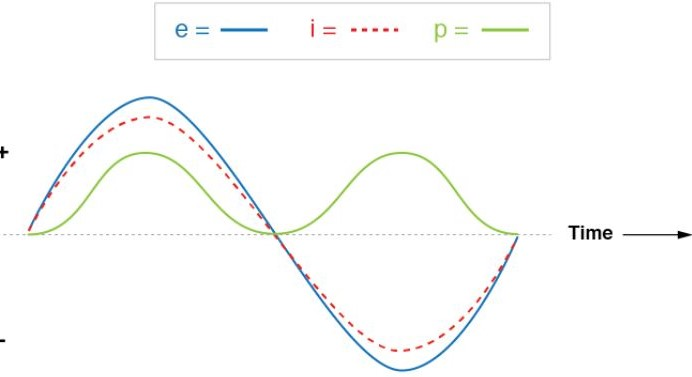# What Is Var In Electrical Engineering? And What’s Its Importance?

Is VAR a useful characteristic of power generation, and how can it be used? What does VAR mean in an electric circuit?

VAR is not useful and can cause significant problems in a circuit. VAR occurs typically in 3 phase power supplies and is known as the reactive power. VAR means Volts Amps Reactive. When you have VAR in a circuit, one phase is lagging, reducing the available power.

## VAR Explained through beer!

Use an easy to understanding analogy. It may seem odd to explain electricity in terms of beer, but this analogy is something that most of you can understand.

When you order a pint of beer, we want a full glass of beer and just a small foam head on the pint.

Most of you would agree the foam is a complete waste and what we want to pay for is the beer section.

Consider that the beer section of the pint is the useful power, the power that can be utilised for, say, driving a motor.

The foam is the apparent power and is entirely useless, but we are still being charged for a full pint of beer even though the top foam part is useless.

## Reactive power defined (VAR)

You can define reactive power as useful power. Reactive power interacts with other components in the electrical system (inductance and capacitance).

In these reactive components, you can consider the current and voltage at 90 electrical degrees apart from each other.

## What creates reactive power?Absorption or capacitors. Reactive power can be absorbed by generators or, in some cases, capacitors which help to maintain a constant voltage.

It can be known as voltage support, but generators can create a lot of heat with voltage support, so its effects are undesirable.

## Why are VARS needed?

To maintain voltage. Reactive power (VARs) is needed to maintain the flow of electrons to deliver active power to the consumer through transmission lines from the power generator.

Motors and other large components require VARs to allow the electrons to flow in the circuit so the motor can spin.

## Do VARs have another name?

Yes, Wattless power. Because the VARs (volts amps reactive) do not energise the component alone, without true power or apparent load.

## Why does inductive load absorb reactive power?

With inductive load, the current drawn by its load lags the voltage, which shows this is always a phase difference between current and voltage.

This shows reactive power is needed in a circuit despite its Wattles status. Reactive power is needed, but it’s not consumed.

## What is the power factor in 3 phase?

The ratio between reactive power and apparent power. The power factor is calculated to discover the apparent power of the reactive power.

If the current and voltage are not lagging, the power factor would be 1. However, in 3 phase circuits, the current and voltage are never in phase creating a power factor of between 0 and 1.

## What is the power factor of single-phase?

The power factor is directly correlated to the AC circuit. The power factor in a single-phase AC circuit is 1. However, DC phases do not have a power factor ratio due to no frequency.

Single-phase resistive circuits usually have a power factor of 0.8, but for ease, it’s considered 1.

## Why is it called reactive power?

It can move in both directions in the circuit. Reactive power can react by moving backwards and forwards in the circuit or reacting upon itself. This is termed reactive power.

## Why is a variable resistor included in a circuit?

To keep current at a constant. Variable resistors can be found in many circuits. They are variable but have an upper value that can not be exceeded.

The variable resistor can adjust the incoming voltage by changing resistance, keeping the current at a constant value.

## What are the advantages of a variable resistor?

Voltage control. In electronics, variable resistors can be found in many circuits where the incoming voltage needs to be ramped down or up depending on the components within the circuit.

Variable resistors regulate voltage and keep current at a constant value.

## When XL XC What is the power factor of an AC circuit?

Possibly zero. When XL=XC, the circuit is considered to be under resonance with a phase angle of 𝚹 being zero.

The resulting circuit reacts live to a purely resistive circuit where the power factor is considered unity.

## Do solar panels generate reactive power?

No, Photons hitting a solar panel generate DC power stored in a battery or directly distributed to a grid.

However, when the AC power is under load, reactive power will be drawn proportional to the power load and the system’s parameters.

## Can batteries provide reactive power?

Yes. The reactive power controls voltage levels and the amount of current flowing in a circuit. The batteries release power when needed, introducing reactive power into the circuit.

Batteries will also absorb reactive power, which will smooth out the capacity issues when using power from a battery.

## Can renewables provide reactive power?

Yes, Renewable energy creates reactive power as the renewables generate electricity by the same means as a power generator except for photovoltaic solar cells.

From wind farms, synchronous generators are used for electricity production, creating reactive power.

## Why is reactive power an imaginary power?

It does not move motors. The imaginary part of the circuit, also known as reactive power, bounces around in the line without the energy being dissipated or converted.

You know it’s there, you can’t see it, and you can’t use it, so it’s called imaginary power.

## Do you get billed for reactive power?

Yes and no, Homeowners do not get billed for reactive power, and if they do, it’s negligible, but industrial users get billed for that part of the power they can’t use.